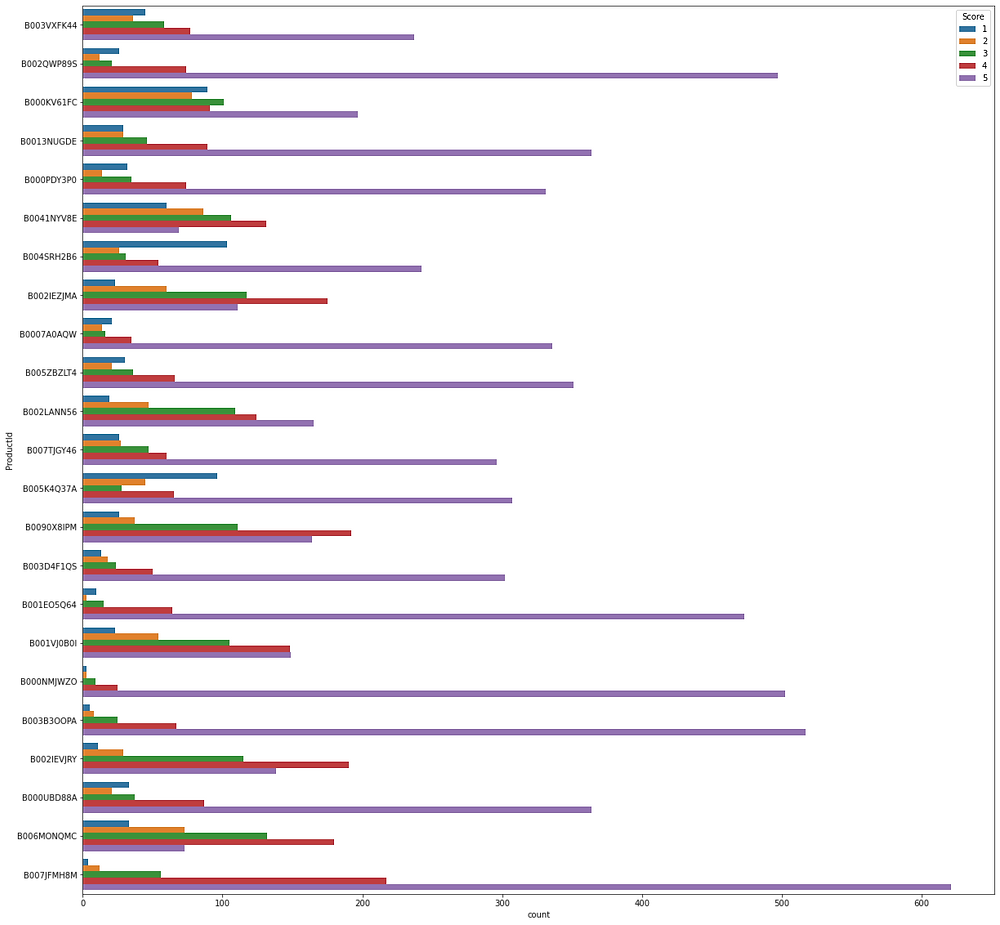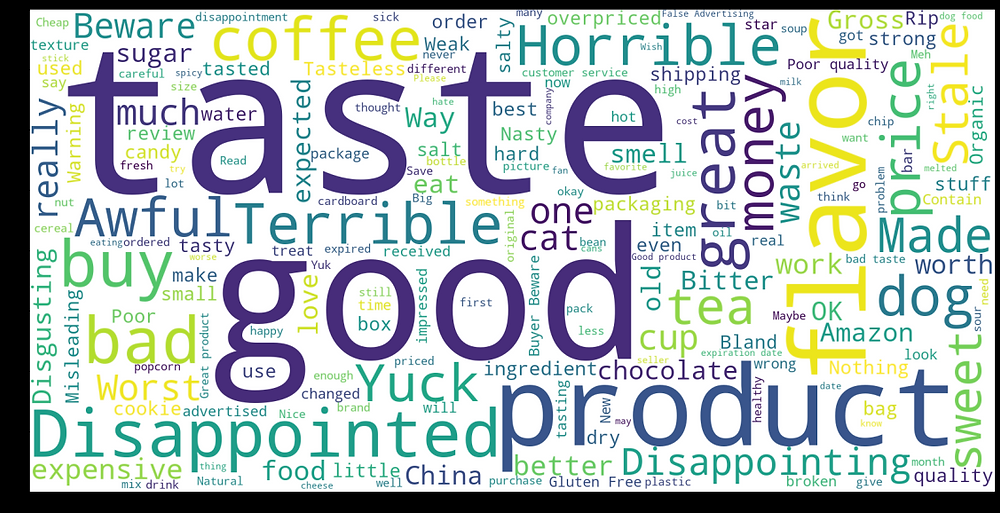Search

# NLP: Text Data Visualization

"Data will talk to you if you are willing to listen."-Jim Bergeson

Text data visualization has many advantages, like getting the most used word at a speed to know what the text is about largely, the number of positive and negative reviews given represented by a graph for all data, user-wise, product-wise, relation between the part of speech, and many more.

Now let us see how to do it. Amazon is a big retail brand and we can see it has a lot of reviews on products. Let's get this data set from Kaggle and get started.

Import few important libraries for the task

```import numpy as np
import pandas as pd
import matplotlib.pyplot as plt
import seaborn as sns

import string
from wordcloud import WordCloud```

Make a data frame from reviews CSV:

`df = pd.read_csv('../input/amazon-fine-food-reviews/Reviews.csv')`

Let's visualize data:

`df.head(10)`Dropping null values if any:

```print(df.shape)
print(df.isnull().values.any())
df.dropna(axis = 0 , inplace = True)
print(df.shape)```

Dropping duplicates:

```df.drop_duplicates(subset=['Score','Text'],keep='first',inplace=True)
print(df.shape)

Visualizing total count of scores:

```plt.figure(figsize=(10,10))
ax = sns.countplot(x=df["Score"],  data=df, order = df["Score"].value_counts().index )
for p, label in zip(ax.patches, df["Score"].value_counts()):
ax.annotate(label, (p.get_x()+0.25, p.get_height()+0.5))```Group by productId

```df.groupby('ProductId').count()
df_products = df.groupby('ProductId').filter(lambda x: len(x) >= 400)
df_product_groups = df_products.groupby('ProductId')

#Count of products and groups
print(len(df_products))
print(len(df_product_groups))```

Plot the scores product-wise

```plt.figure(figsize=(20,20))
sns.countplot(y="ProductId",  hue="Score", data=df_products);```Group by UserId who gave more than 100 reviews:

```df.groupby('UserId').count()

df_users = df.groupby('UserId').filter(lambda x: len(x) >= 100)
df_userGroup = df_users.groupby('UserId')
print("Number of Users:"+ str(len(df_userGroup)))
df_products = df_users.groupby('ProductId')
print("Number of products:"+ str(len(df_products)))```

Plotting users as per their given score ratings :Now let's see which are the words used mostly in positive reviews and the most used words in negative reviews.

For this import library for data cleaning:

```from nltk.tokenize import word_tokenize
from nltk.tokenize import sent_tokenize
from nltk.stem import WordNetLemmatizer
from nltk.corpus import stopwords```

Make functions for removal of stopwords, lemmatizing and cleaning text:

```def remove_Stopwords(text ):
stop_words = set(stopwords.words('english'))
words = word_tokenize( text.lower() )
sentence = [w for w in words if not w in stop_words]
return " ".join(sentence)

def lemmatize_text(text):
wordlist=[]
lemmatizer = WordNetLemmatizer()
sentences=sent_tokenize(text)
for sentence in sentences:
words=word_tokenize(sentence)
for word in words:
wordlist.append(lemmatizer.lemmatize(word))
return ' '.join(wordlist)

def clean_text(text ):
delete_dict = {sp_character: '' for sp_character in string.punctuation}
delete_dict[' '] = ' '
table = str.maketrans(delete_dict)
text1 = text.translate(table)
textArr= text1.split()
text2 = ' '.join([w for w in textArr])

return text2.lower()```

Segregate positive and negative reviews:

```mask = (df["Score"] == 1) | (df["Score"] == 2)
mask = (df["Score"]==4) | (df["Score"]==5) | (df["Score"]==3)
print(len(df_rating1))
print(len(df_rating2))```

Cleaning the text of stopwords, lemmatizing, and cleaning punctuations:

```df_rating1['Text'] = df_rating1['Text'].apply(clean_text)
df_rating1['Text'] = df_rating1['Text'].apply(remove_Stopwords)
df_rating1['Text'] = df_rating1['Text'].apply(lemmatize_text)

df_rating2['Text'] = df_rating2['Text'].apply(clean_text)
df_rating2['Text'] = df_rating2['Text'].apply(remove_Stopwords)
df_rating2['Text'] = df_rating2['Text'].apply(lemmatize_text)

df_rating1['Num_words_text'] = df_rating1['Text'].apply(lambda x:len(str(x).split()))
df_rating2['Num_words_text'] = df_rating2['Text'].apply(lambda x:len(str(x).split()))```

WordCloud view of negative reviews:

```wordcloud = WordCloud(background_color="white",width=1600, height=800).generate(' '.join(df_rating1['Summary'].tolist()))
plt.figure( figsize=(20,10), facecolor='k')
plt.imshow(wordcloud)```WordCloud view of positive reviews:

```wordcloud = WordCloud(background_color="white",width=1600, height=800).generate(' '.join(df_rating2['Summary'].tolist()))
plt.figure( figsize=(20,10), facecolor='k')
plt.imshow(wordcloud)
plt.axis("off")

```Let us see how to visualize the relation between parts of speech.

For this import spacy

```import spacy
from spacy import displacy
doc=nlp(u'The blue pen was over the oval table.')```

Visualize as below:

`displacy.render(doc, style='dep')`Now let's fill in few colors to this representation with some options:

```doc1=nlp(u'I am Namrata Kapoor and I love NLP.')
options={'distance':110,'compact':'True', 'color':'white','bg':'#FF5733','font':'Times'}
displacy.render(doc1, style='dep',options=options)```Conclusion

We see here a few of the techniques of text visualization with the use of WordCloud, SNS, and matplotlib.

There are more things that can be explored once sentiment analysis is used on it and we dig in deeper with some rules that define clearly if the review was given for product or delivery.

Also, stopwords like 'not' change the meaning of a word which has to be catered to by replacing them with the antonyms before applying any stop words and visualizing in wordcloud.

These are a few of the things that can optimize what I did.